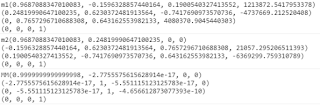# Transform Method to get inverseMatrix4 has a low precision!?

Today,I use the method “Cesium.Matrix4.inverseTransformation” to get the inverse matrix,and use the method “Cesium.Matrix4.multiplyTransformation” to check the result,then I get the result below:m1 is a basic matrix4,and the m2 is the inverse matrix4,MM is the result of m1 multiply by m2,the accurate result must be identity matrix4.But in the picture,we can found it is a little different,Will it cause some problems?I really want to know it.

Hello,
I think it’s due to limitations in IEEE 754 format (Double-precision floating-point format). It’s a compromise between speed execution and precision.
For example, test these codes:

1. Math.cos(0.0000001)
2. Math.cos(0.00000001)

Thank you for your reply, I follow you to the code test,and found the difference.The compromise on the cesium would seem to cause some problems,like transforming the model from model frame to world frame.I don’t know how deep is the influence.